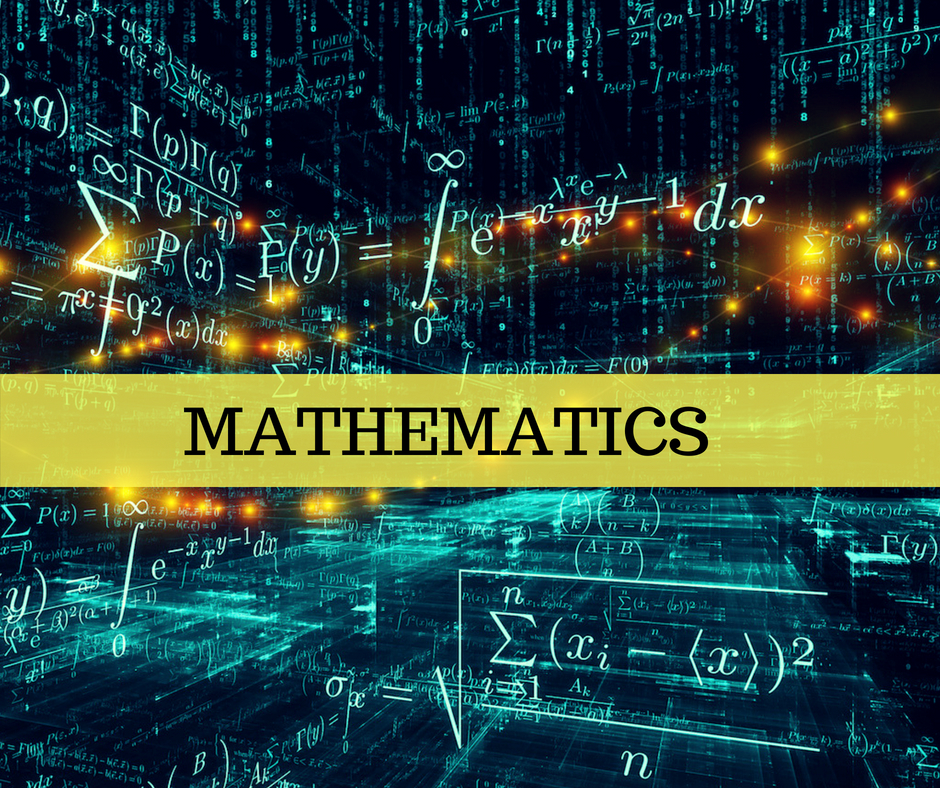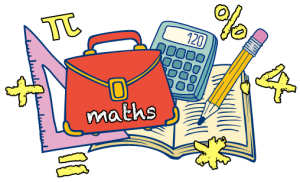Today’s generation Mathematics is very important to understand the different subject.  If you have the grip on mathematics then you can gain any subject easily. It has no definition this is only understood, a good mathematics tricks as a formal area of education and knowledge was created about 5,000 years ago.

Mathematics is a subject which is full of logic and full of confusion. Only “Math Practice Makes Math Perfect”. There is no Alternative Solution.

Math’s is not about solutions, it’s about the method. the field of Mathematics and the requirements to study it as an international student.  How to study math’s is the main problem for every student and every teacher don’t understand how to make understanding about math’s.Maths Tutors & Teachers are not always helpful to you, this may not give you the best result till you will not able to understand yourself. It has  so many difficulties and easiest answer; more we think math’s is complicated the more we get complicated. In Mathematics to learn is to learn formulas very nicely and quickly and practice it daily without any laziness.some student do laziness because of this sum is lengthy and tough too we will do in the exam but no incorrect. Do practice daily it will be made you remember how to solve the sum so easily and at speedy. Math’s subject is very simple if we think to solve it and have courage and hope too for doing the sums at the nice level then everything is possible.

### Tips to get nice marks in mathematics are very simple :

1. Read and memorize the formula as you can do.
2. Make your own notes for doing sums.
3. Practice for more sum related to the same topics.
4. Be on more focus, where mistakes are very much.
5. Don’t revise the simple sums and get tough sums practice.

That’s all the tips are and at last, I want to say one thing that mathematics is the very simple subject about all and this subject can make a huge difference with your mental ability and brain power.